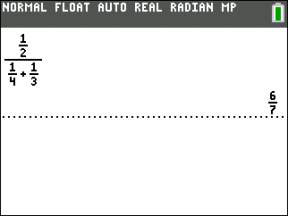##### TI-84 Plus CE Graphing Calculator For Dummies, 3rd EditionThere’s no fraction key on the TI-84 Plus calculator, per se, but many fraction tools are built into this calculator. For starters, isn’t a fraction just division in disguise? So, pressing / between two numbers creates a fraction.

## Setting the mode

Do you prefer fractions or decimals? Would you rather work with an improper fraction or a mixed number? There’s no right answer to these questions, but what would make you (or your teacher) happy? Changing the mode of your calculator forces the calculated answers into a form of your liking. Be careful; this is a big decision on your part!

Setting the mode not only affects calculations on the Home screen, but also the way lists and sequences are displayed.

To change the form of your calculated answers, press [MODE]. Use the arrow keys to scroll to the 11th line, ANSWERS. Here, there are three choices that affect how calculated answers are displayed:

• AUTO: Choosing AUTO displays the answers in a similar format to the way the expressions are entered. If the expression contains a decimal, then you should expect the answer to be in decimal form. If the expression is entered in fraction form, then you should expect the answer to be expressed in fraction form. See the first screen.

• DEC: The DEC mode forces the answers to be displayed as decimals. See the second screen.

• FRAC — APPROX: When possible, the FRAC — APPROX mode displays answers as fractions. See the third screen.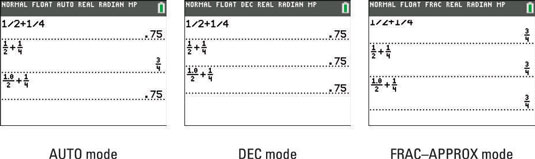There’s one more mode decision you need to make. What type of fraction do you prefer: improper fractions or mixed numbers? Press [MODE] and change the FRACTION TYPE to one of these two choices:

• n/d: Fractions are displayed in simplified fraction form.

The numerator of a fraction must contain less than seven digits and the denominator of a fraction must not exceed 9999.

• Un/d: When possible, fractions are displayed as a mixed number.

To avoid errors and potential problems, enter U, n, and d as integers with a maximum of three digits.

## Converting fractions and decimals

There’s an easy way to convert a decimal to a fraction, regardless of the mode setting. You can access the Frac and Dec functions in the first two options in the Math menu. The Frac function displays an answer as a fraction.

Type the expression and press [MATH][ENTER][ENTER] to display the expression as a fraction. Press [MATH][ENTER][ENTER], and your answer is converted to a fraction. See the first screen. If your calculator can’t convert an expression to a fraction, it lets you know by redisplaying the decimal.

How do you convert an infinite repeating decimal into a fraction? Just type at least ten digits of the repeating decimal and press [MATH][ENTER][ENTER]. See the second screen.

The Dec function converts a fraction to a decimal. Enter the fraction and press [MATH][ENTER]. Of course, if you’re not thinking ahead and your answer is in fraction form, just press [MATH][ENTER] to display your answer as a decimal. An example is shown in the third screen.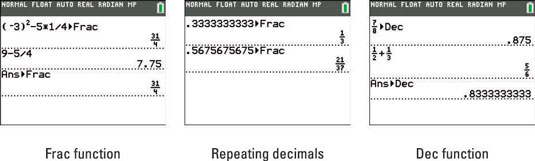The MTRX menu can only be accessed by pressing [ALPHA][ZOOM] to access the MTRX shortcut menu. However, the rest of the shortcut menus can also be accessed by standard menus. For example, the FRAC menu can also be accessed in two places in the MATH menu. Press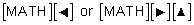(at the bottom of the NUM menu) to find the FRAC menu in a standard menu. See the second and third screens.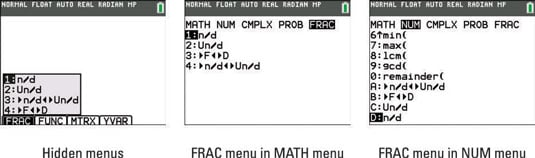## Entering fractions and mixed numbers

Press [ALPHA][Y=] to access the FRAC menu. The first two options in the FRAC menu are easy-to-use fraction templates:

• n/d: Enter fractions in the fraction template.

• Un/d: Enter fractions in the mixed number template. See the first screen.

The next two options are used for conversion: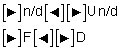• Converts a mixed number to an improper fraction, or an improper fraction to a mixed number. See the second screen.

• Converts a fraction to a decimal, or vice versa. See the third screen.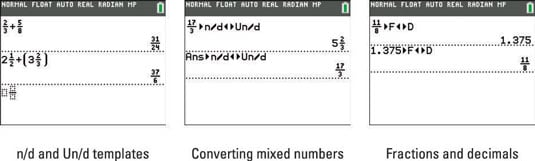## Entering complex numbers in fractions

The n/d fraction template has its limitations. Complex numbers may not be used in the n/d fraction template. To enter the complex number, i, press [ALPHA][.]. Entering a complex number in the n/d fraction template produces an error message as seen in the first screen.

Don’t worry! You can enter complex numbers into fractions the old-fashioned way, using parentheses and the division key. Your calculator automatically simplifies fractions that contain a complex number in the denominator. See the second screen.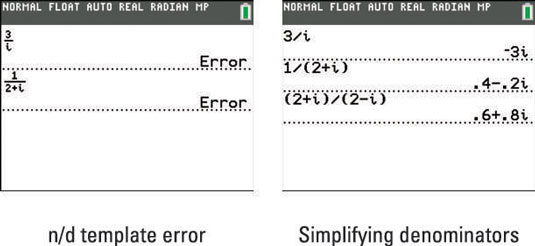## Entering complex fractions

Complex fractions are fractions that contain one or more fractions in the numerator or denominator. In other words, complex fractions have fractions inside of fractions. No problem. Complex fractions can easily be entered in your calculator by using the n/d fraction template multiple times in the same fraction.

Press [ALPHA][Y=] to access the n/d fraction template.